Tom Kelliher, CS26

Nov. 26, 1996

# Number Representation

Properties of number systems:

1. Positional.

2. Weighted.

Example: decimal

Binary weights:

1. Integer.

2. Fraction.

3. Binary point.

4. Bit numbering.

Unsigned value of an binary integer:Representation error: splitting the gap.

## Sign-and-Magnitude Representation

Msb is the sign bit:

1. Has no weight.

2. If 0, the number is ``positive.''

3. If 1, the number is ``negative.''

4. Value: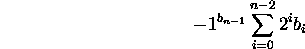5. Problems:
1. Multiple representations of 0.

2. Hard to build good adders.

6. Decimal-to-binary conversions. Vice versa.

7. Symmetric range.

## 1's-Complement Representation

Msb is the sign bit:

1. Has negative weight: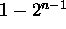.

2. If 0, the number is ``positive.''

3. If 1, the number is ``negative.''

4. Value: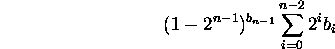5. Problems:
1. Multiple representations of 0.

2. Wrap-around carrys.

6. Conversions: decimal, binary, negative, positive.

7. Symmetric range.

## 2's-Complement Representation

Msb is the sign bit:

1. Has negative weight: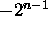.

2. If 0, the number is ``positive.''

3. If 1, the number is ``negative.''

4. Value: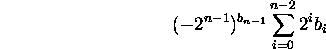5. Problems:
1. Asymmetric range.

6. Conversions: decimal, binary, negative, positive.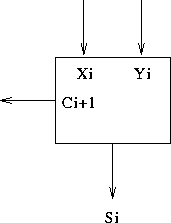Truth table: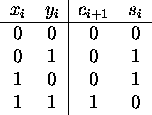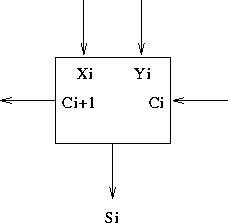Truth table: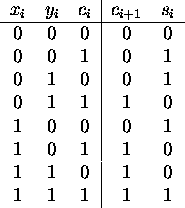Delay model for a full adder:

1. Two gate delays from any input to any output.

1. How would you tie full adders together to form an n-bit adder?

2. What would it's delay be?

3. For eight bit adders, what is an example input that would cause a full precision carry?

1. Why bother with speeding up addition?

2. Can we pre-compute the carries?

3. Carry generate:.

4. Carry propagate: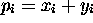.

5. Some carry equations:
1.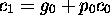2.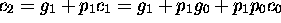3.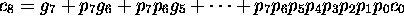6. A bit of recursion:
1. Base case: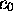2. Recursive step:7. Why this isn't feasible.

Block diagram:Block generate, propagate.

2. What is the fan-in?

3. What is the delay model from inputs to outputs?

1. Design a 4-Group carry lookahead unit.

Block diagram: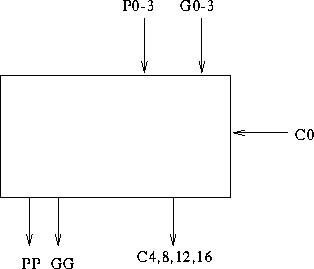Use of block generates, propagates.

2. What is the fan-in?

3. What is the delay model from inputs to outputs?